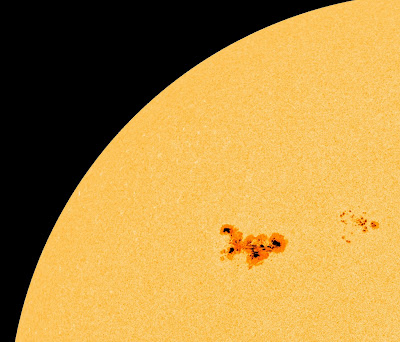## Wednesday, March 7, 2012The news was released this morning that an "S-3 class" solar radiation event has been triggered by a monster flare that occurred yesterday. The giant flare (see top graphic from spaceweather.com) is associated with a giant delta class sunpot (see middle graphic) that is expected to generate a number of X-class flares.

Flares generally are headed under three categories by their energies: C, M and X. The X class is the major flare category since those events (delivering x-radiation) can trigger long-lasting storms affecting the ionosphere (among which sudden ionospheric disturbances and short wave fadeouts and blackouts are numbered) as well as interference with commercial aircraft radar. Thus, we always pay special attention to these events in flare forecasts.

Interestingly, the actual flare eruption also incepted a coronal mass ejection, shown here in a movie from the Solar and Heliospheric Observatory. Analysts examining the film and other directional data believe this will only strike us a "glancing blow" - possibly generating enormous auroral displays over the impact dates (Mar. 8-9).

How is it that such giant spot as 1429 get so cranked up energetically that they are able to release so much energy? One very plausible model is depicted in the lowest graphic in which I show two coronal loops - with their bases or "footpoints" putatively anchored in a complex large sunspot such as 1429 would represent. As the photospheric motions increase the loops wrap around each other exchanging magnetic helicity (H(r)) and setting the stage for a fantastic eruption.

Magnetic helicity” was probably first introduced by K. Moffat in the late 1950s as a topological invariant that describes the complexity of a magnetic field. Generally, magnetic helicity also has “twist” and “writhe” components. It is written as a function of the vector potential (A) and the magnetic field (B), and measures the topological linkage of magnetic fluxes (φ).

The magnetic helicity H of a field with characteristic magnetic induction B within a volume V is defined:

H = INT_ V A*B dV

where INT denotes integral over the volume V .

In actual working solar conditions, one prefers what's called a "gauge-invariant form of H" and this is provided by the “relative helicity” – wherein one subtracts the helicity of some reference field (B (o), e.g. associated with the force-free parameter alpha = 0) and having the same distribution of the normal component of B on the surface (S). Thus:

H_r = [INT _V A*B dV - INT V_o A_ o B_o dV_o]

It is hypothesized that shearing and twisting of the field “injects” helicity and that this may be useful in quantifying: a) how much magnetic free energy becomes available, and b) whether instability can be predicted based on observed indicators of helicity at the level of the photosphere-chromosphere.H(R) can then be resolved into two components such that:

d H(R)/ dt = d H(R) [T] / dt + d H(R) [W] / dt

where term 1 on the RHS refers to the “twist” and term 2 to the “writhe”. We see evidence of the Sun’s magnetic helicity in the solar corona as well as the solar wind that streams past Earth. In eruptive prominences, for example, we actually have images that show the twist and writhe (or helical structure) associated with topological helicity – and which is magnetic helicity in the Sun’s magnetic environment.

For example, if you carefully inspect and study the prominence in the upper right of the image below, you can discern both twist and writhe in the plasma filaments.:
http://antwrp.gsfc.nasa.gov/apod/ap060416.html

Evidently then, prominences are capable of transporting magnetic helicity in the solar corona.

Warning! Specialized stuff!

We now get to the lowest graphic and how that energy is quantified by mutual twisting. Thus, the two different loops shown (a1-a2 and b1-b2) will conform to motions and transfer of relative helicities such that:

d H(a1-a2)/ dt = d H(r’ ) [Ta1a2] / dt = d/dt [ (L1 B_Θ(a))/ (a B z (a))]

d H(b1-b2)/ dt = d H(r ) [Tb1b2] / dt = d/dt [ (L2 B_Θ(b))/ (b B z (b))]

and: L1 > L2

with the radius for L1 = a and the radius for L2 = b, each of which is assumed to remained constant. Thus, the respective aspect ratios (ratio of length of loop to radius) are: L1/a and L2/b.

When a collision occurs between the loops, we expect:

d H(a1-a2)/ dt + d H(b1-b2)/ dt =

d/dt [ (L1 B_Θ(a))/ (a B z (a))] + d/dt [ (L2 B_Θ(b))/ (b B z (b))]

and the increase in relative velocity in terms of the linear velocities (projected on the axes: +x, -x) is:

d/dt [L1 cos w t] = - w L1 sin w t and d/dt [-L2 cos w t] = w L2 sin w t’

where we use w for the angular velocity (the Greek common omega symbol in the graphic)

therefore:

delta H(r’) [Ta1a2] + delat H(r) [Tb1b2] = - w L1 sin w t + w L2 sin w t’

Of interest for generating large flares (hence significant CMEs) is that the relative twists be asynchronous - which may occur if one of the loops or a segment thereof alters its length during the mutual winding. If on the other hand, the relative rotary motions of each loop L1 and L2 are synchronous, w t = w t’ = 2π, one obtains: 2 rho sin (2 w t - w t’ )/[ π (a + b)/2)^2] ~ 0.

In other words there is essentially no net change in helicity. This is more likely to be true for a line-tied case, and the zero net change means an instability is unlikely.

Whether one understands the preceding math or not, we might be in for a nice display in the skies over March 8-9.